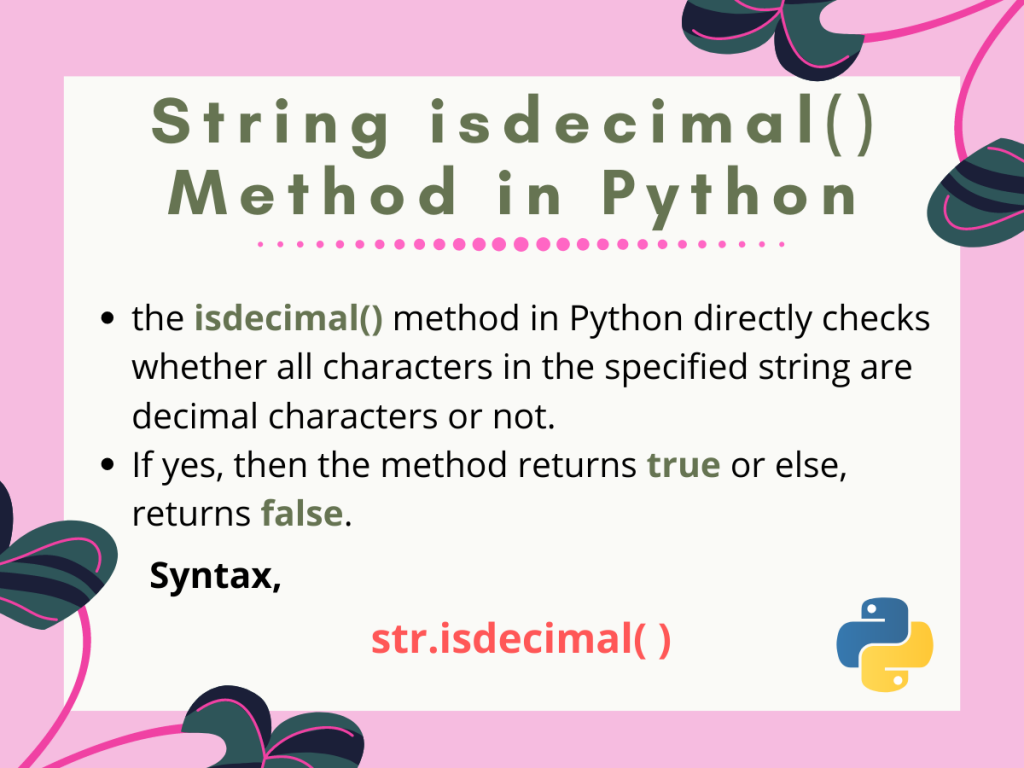# The Python isdecimal() Method## Introduction

We’ll be introducing the python isdecimal() method in this tutorial. What if a user wants to check whether a given string contains a number or more specifically a decimal number or not? That can be a case when the user needs to convert the string to any other number types like integer or float or etc..

As a solution to this problem, Python comes with a built-in checking method, `isdecimal()`. Let us dig into the method and consequently understand how the method works and can be used accordingly.

## Python isdecimal() Method Basics

In general, the Python `isdecimal()` method directly checks whether all characters in the specified string are decimal characters or not. If yes, then the method returns `true` or else, returns `false`.

### Syntax for Python isdecimal() method

Let us look at the syntax for the Python `isdecimal()` method, following which we can use the method.

```status = str.isdecimal( )
```

Here:

• `str` is the string for which we are checking if it comprises of only decimal characters or not
• `status` stores the value returned by the `isdecimal()` method. That is true for all decimal characters or else, false.

Note: Here, status just holds either true or false, hence the type of status is `bool`.

## Basic example for the isdecimal() method

So here is a small example, which explains how to use the `isdecimal()` method for strings in Python.

```string1="1010"
print("String is: ",string1)
print("Result: ",string1.isdecimal())

string2="a10101"
print("String is: ",string2)
print("Result: ",string2.isdecimal())
```

Output:

```String is:  1010
Result:  True
String is:  a10101
Result:  False
```

Here:

• String1 consists of all decimal characters. Hence the `string1.isdecimal()` statement returns a `True` result
• Whereas, string2 has a character ‘a’ in it. Due to the fact that string2 doesn’t comprise of all decimal characters, the result, in this case, is `False`.

Note: The `isdecimal()` method in Python does not consider fractions, subscripts, superscripts, or any other Unicode implemented characters as a decimal or digit characters. Hence, for the presence of any of the numeric characters in a string, the method returns `False`.

## How Python isdecimal() Works

The code snippet below illustrates how the `isdecimal()` method in Python for strings works and what it returns.

```# . is not considered as decimal character
string1="55.55"
print("String is: ",string1)
print("Result: ",string1.isdecimal())

#Pure character string
print("String is: ",string2)
print("Result: ",string2.isdecimal())

#U+2082 unicode for subscript 2
string3="222\u2082"
print("String is: ",string3)
print("Result: ",string3.isdecimal())

#U+2074 unicode for superscript 4
string4="34\u2074"
print("String is: ",string4)
print("Result: ",string4.isdecimal())

#U+2152unicode for 1/10 fraction
string5="10\u2152"
print("String is: ",string5)
print("Result: ",string5.isdecimal())

#@ not considered as decimal character
string6="@1234"
print("String is: ",string6)
print("Result: ",string6.isdecimal())
```

Output:

• For string1, the `isdecimal()` method returns a `false` value because of the dot(.) character present in between the digits. The method considers it not to be a decimal character
• Again for string2, the string completely or purely consists of characters and hence the method returns a `false` result
• For all the strings, string3, string4 and string5. The `isdecimal()` method returns `false` because all of them contain Unicode values corresponding to non-digit numerical characters
• At last for string6 too, the method returns a `false` because of the fact that the string contains the ‘@‘ character, which again is a non-decimal character.
So, in this tutorial, we learned about the built-in string `isdecimal()` method in Python, how it is used as well as how it works. For any questions feel free to comment below.